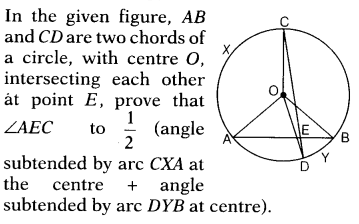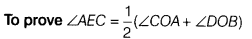# Given figure, AB and CD are two chords of a circle, with centre O, intersecting each other at point EGiven AB and CD are two chords of circle with centre O, which intersects at E.Construction Join AC, BC and BD.
Proof AC is a chord.
∴ ∠AOC=2∠ABC…(i)
[angle subtended at centre is double the angle subtended at circumference]
Similarly, ∠DOB = 2DCB… (ii)
[angle subtended by the chord BD]

On adding Eqs. (i) and (ii),
we get ∠AOC + ∠DOB = 2(∠ABC + ∠DCB) …(iii)
In ∆CEB,∠AEC = ∠ECB + ∠CBE
[exterior angle is equal to the sum of two opposite interior angles ]
∠AEC = ∠DCB +∠ABC ,…(iv)
[∴ ∠ECB =∠DCB and ∠CBE ∠ABC] :.

From Eqs. (iii) and (iv), we get
∠AOC + ∠DOB =2∠AEC